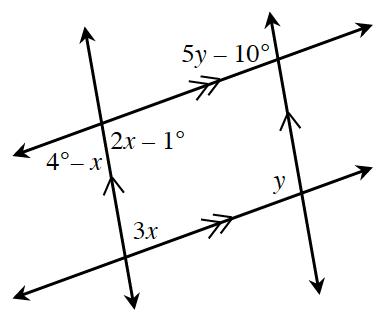### Home > CCG > Chapter 8 > Lesson 8.1.2 > Problem8-23

8-23.

Multiple Choice: Which equation below is not a correct statement based on the information in the diagram?

1. $3x + y = 180°$

1. $2x-1°=4°-x$

1. $2x-1°=5y-10°$

1. $2x-1°+3x=180°$

Read the Math Notes box in Lessons 2.1.1 and 2.1.4 about angle pair relationships.

B because $2x-1º$ and $4º-x$ are a linear pair so $2x-1º+4°-x=180$, not $2x-1º=4º-x$.